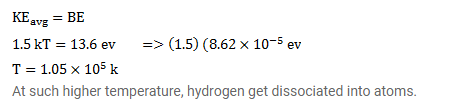# The average kinetic energy of molecules in a gas at temperature

Question:

The average kinetic energy of molecules in a gas at temperature $t$ is $1.5 \mathrm{kT}$. Find the temperature at which the average kinetic energy of the molecules of hydrogen equals the binding energy of its atoms. Will hydrogen remain in molecular form at this temperature? Take $\mathrm{k}=8.62 \times 10^{-6} \mathrm{eV} / \mathrm{K}$.

Solution: